# Excel AVERAGE & MEDIAN FunctionIn this tutorial, we will learn about How People use AVERAGE to fool you. Use MEDIAN in Excel with the help of 2 Formulas given below.

1. AVERAGE Function in Excel
2. MEDIAN Function in Excel

Case 1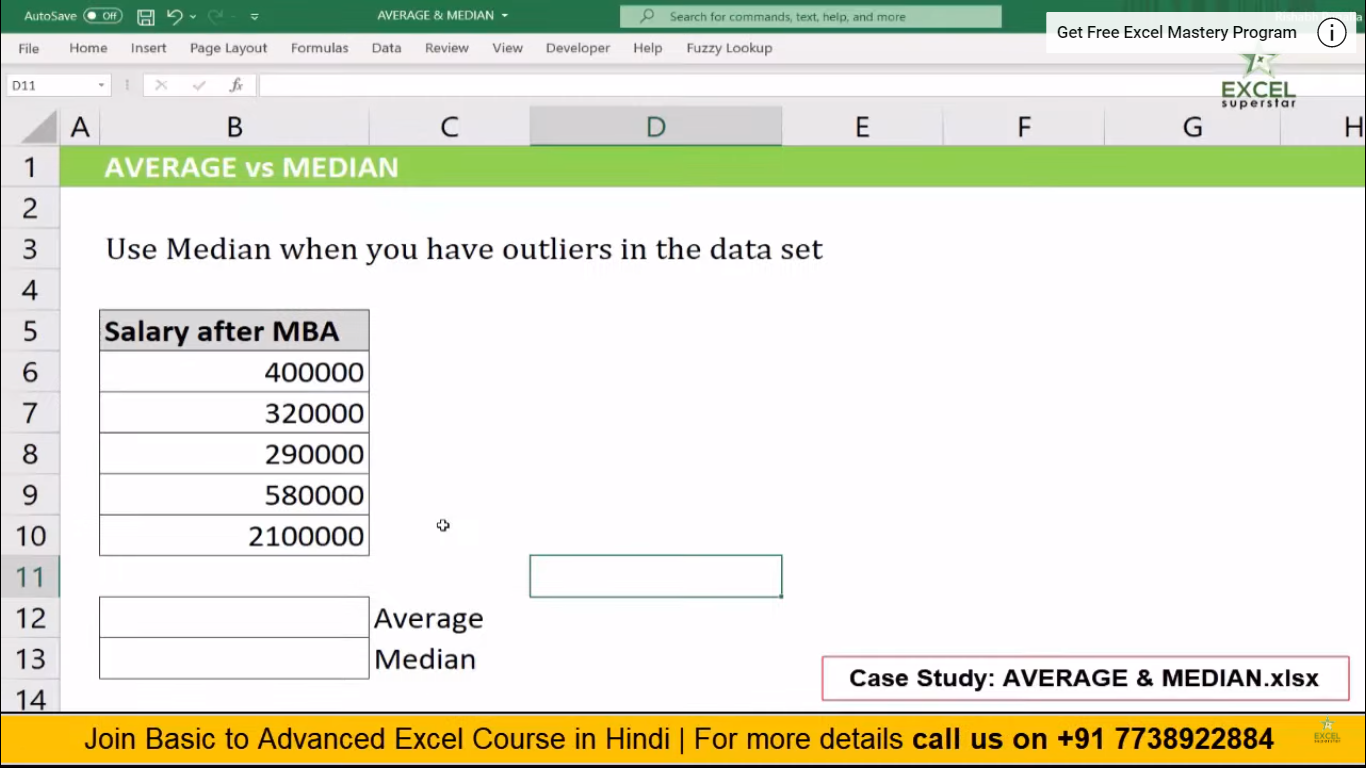In this data, I have one column named Salary after MBA, and inside there are various salaries in each row.

Now I want to find out the average salary after MBA from the given data. So to find out the average salary we will be using the Average Formula.

1. Press =AVERAGE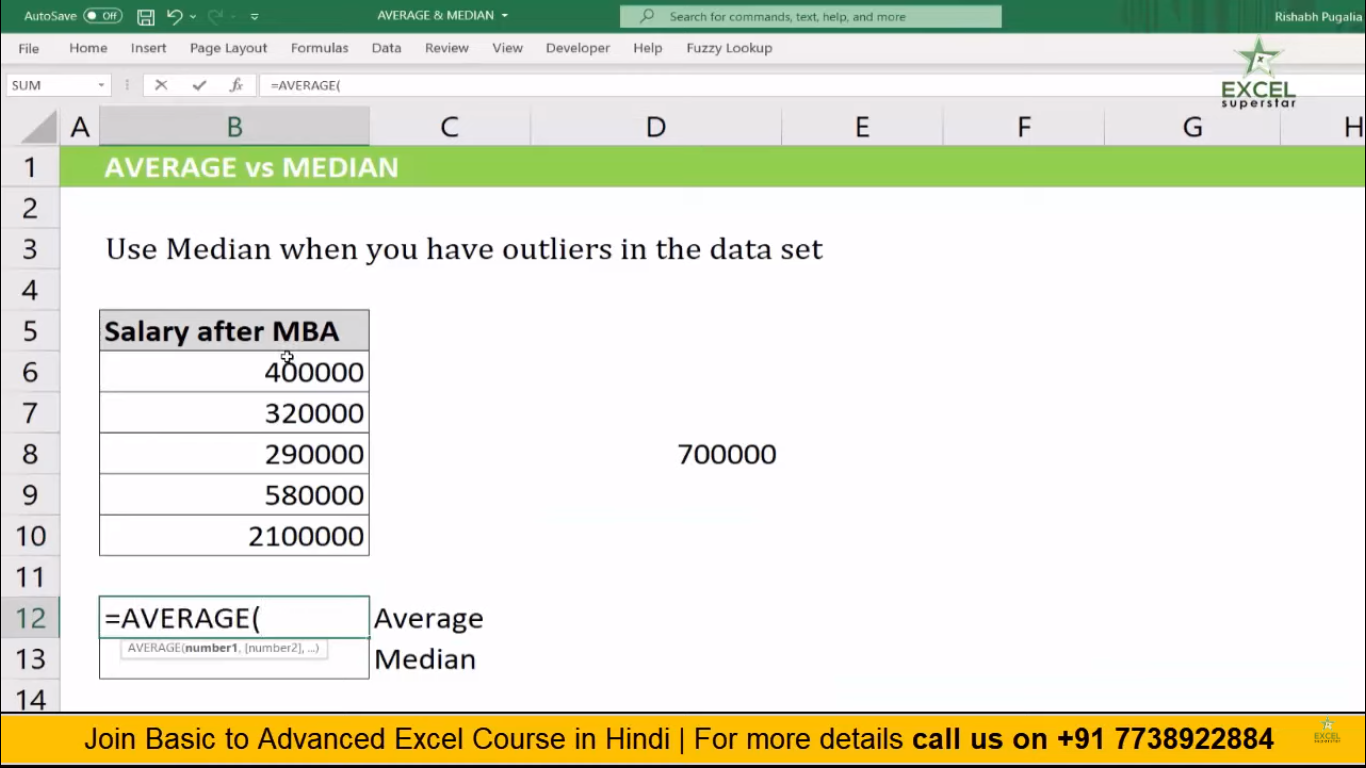2. Choose the entire data =AVERAGE(B6:B10)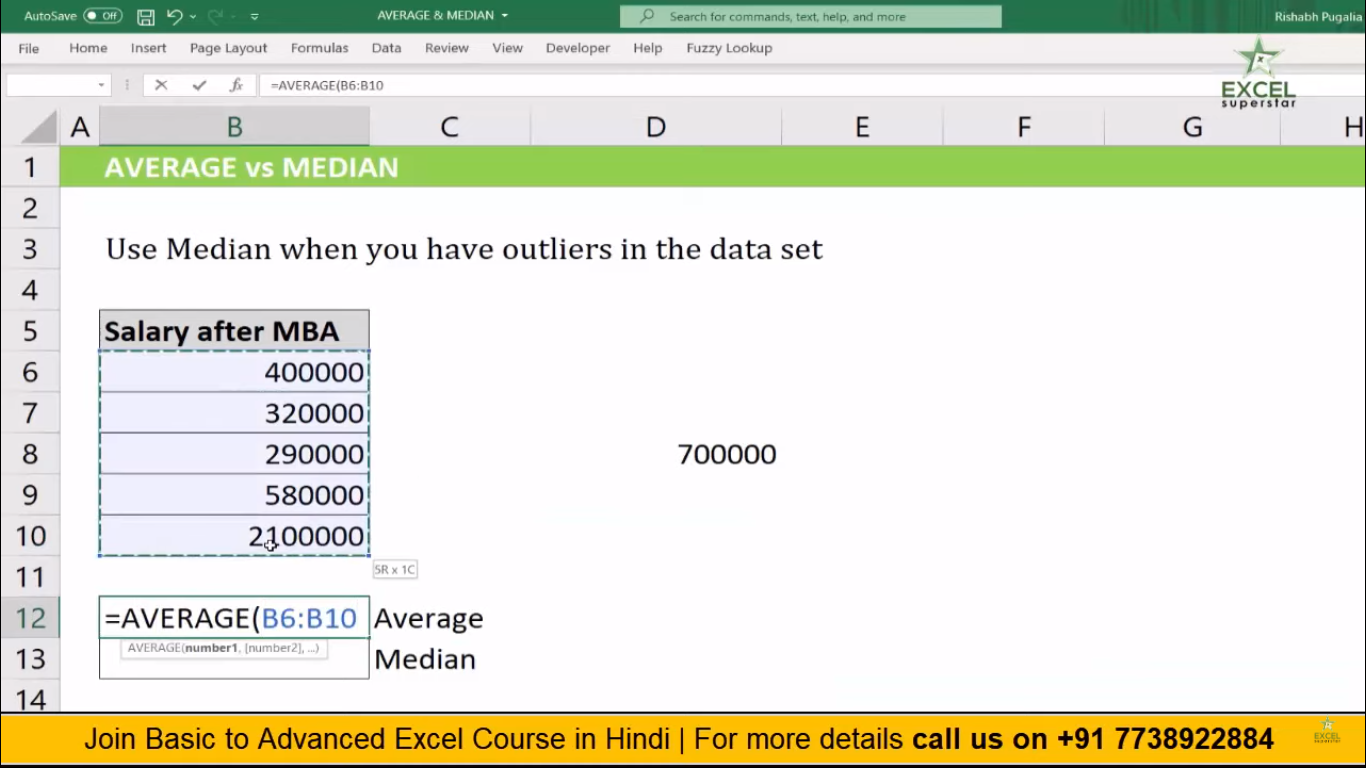3. Press Enter and the answer is RS 738000 which is the expected salary after MBA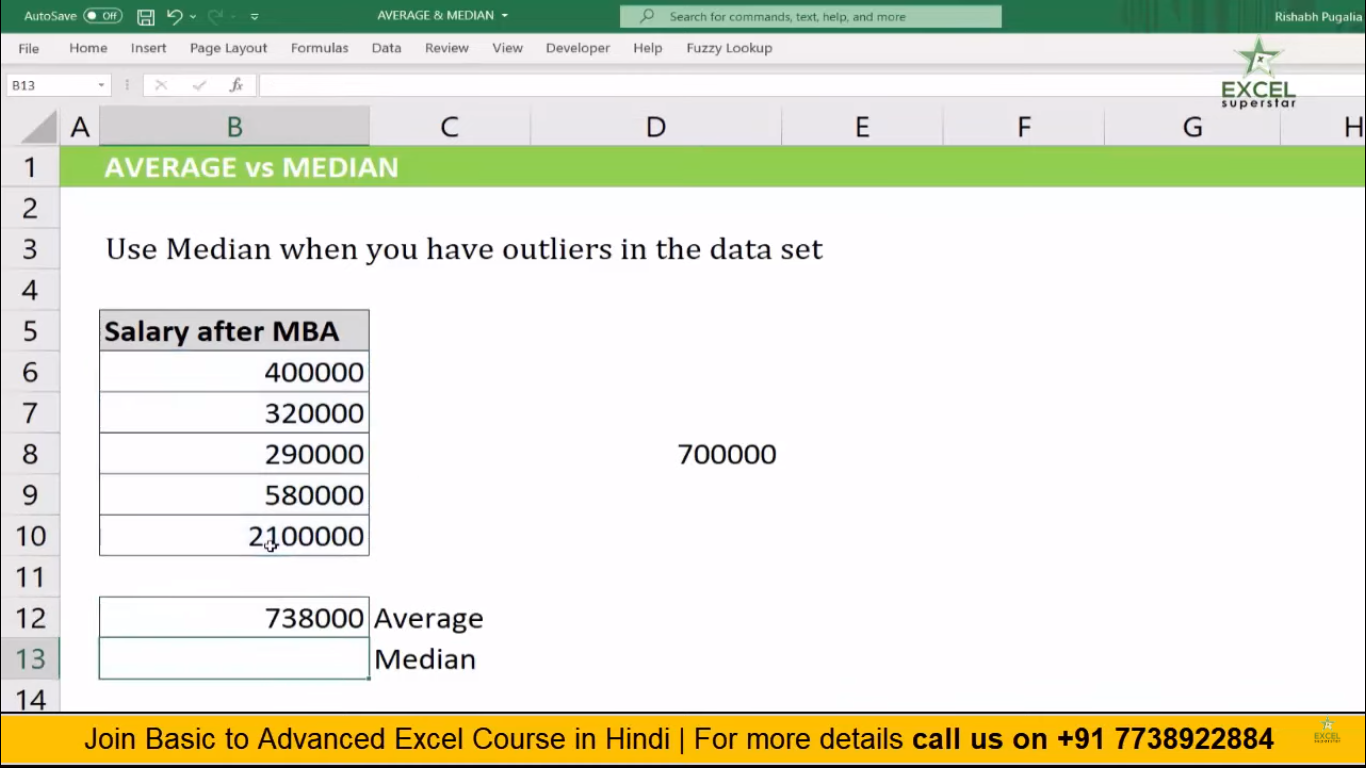Case 2In this given data, one person is a US citizen, so when the person migrates to his home country, then the salary will be high according to purchasing power.

So when there are outliers in the table, and you want to know the Average salary after MBA, then you will be using Median Formula by following the steps given below:

1. Press =MEDIAN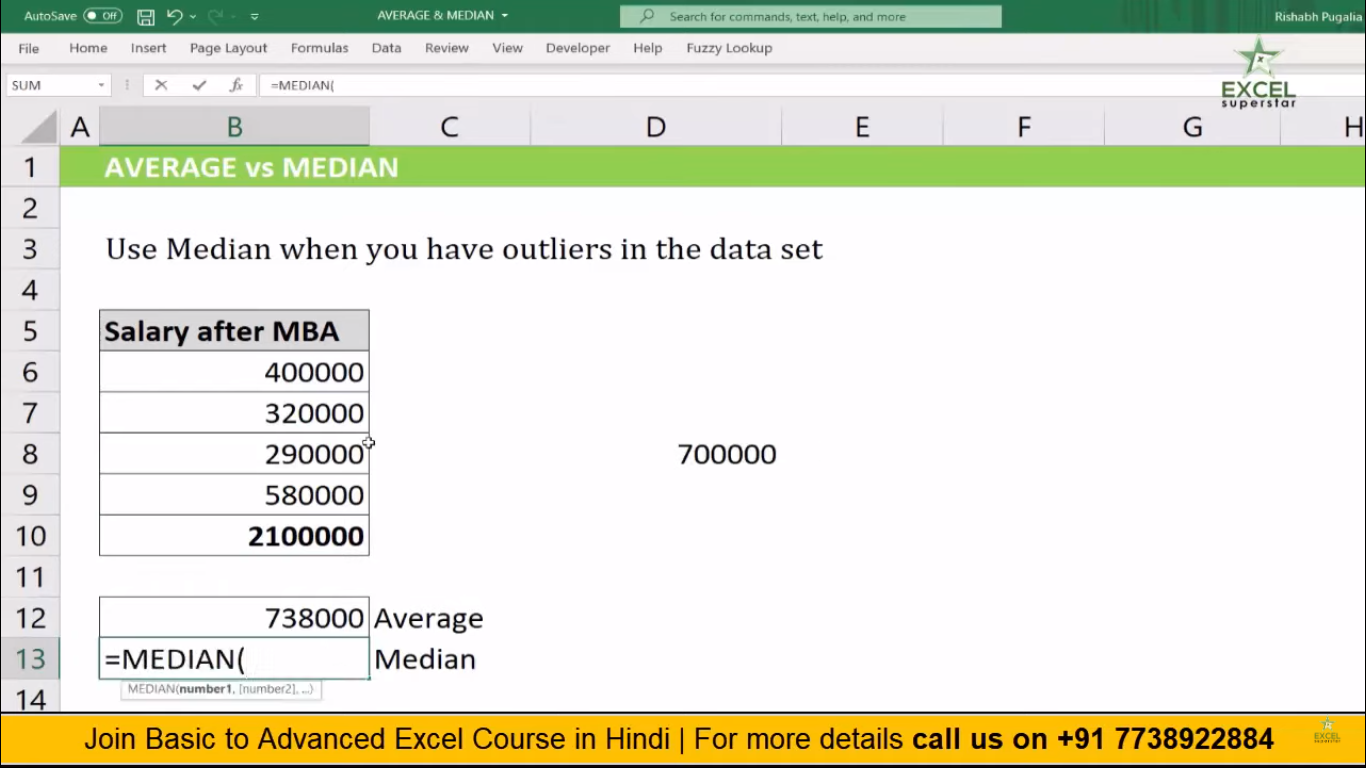2. Choose the entire data =MEDIAN(B6:B10)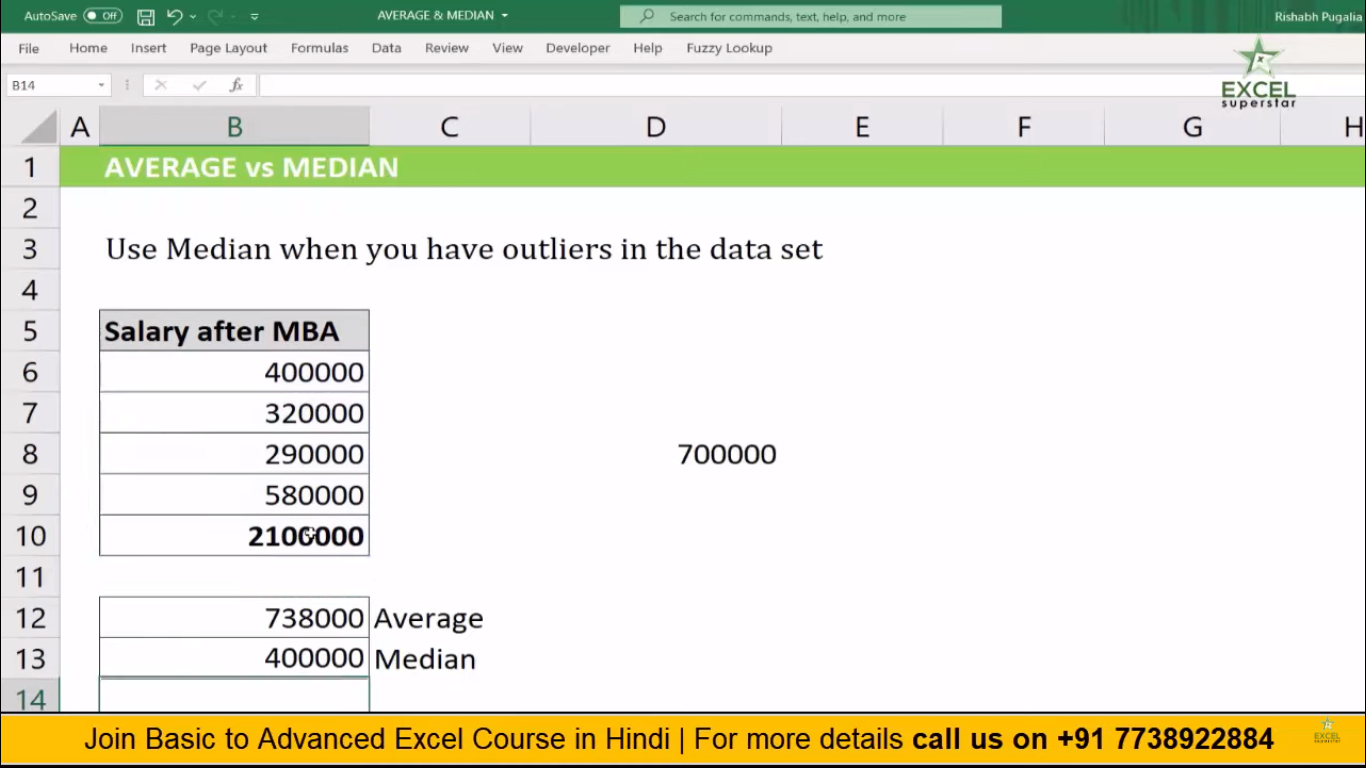3. Press Enter and the answer is 400000 which means most of the people will get this much amount as an expected Salary after MBA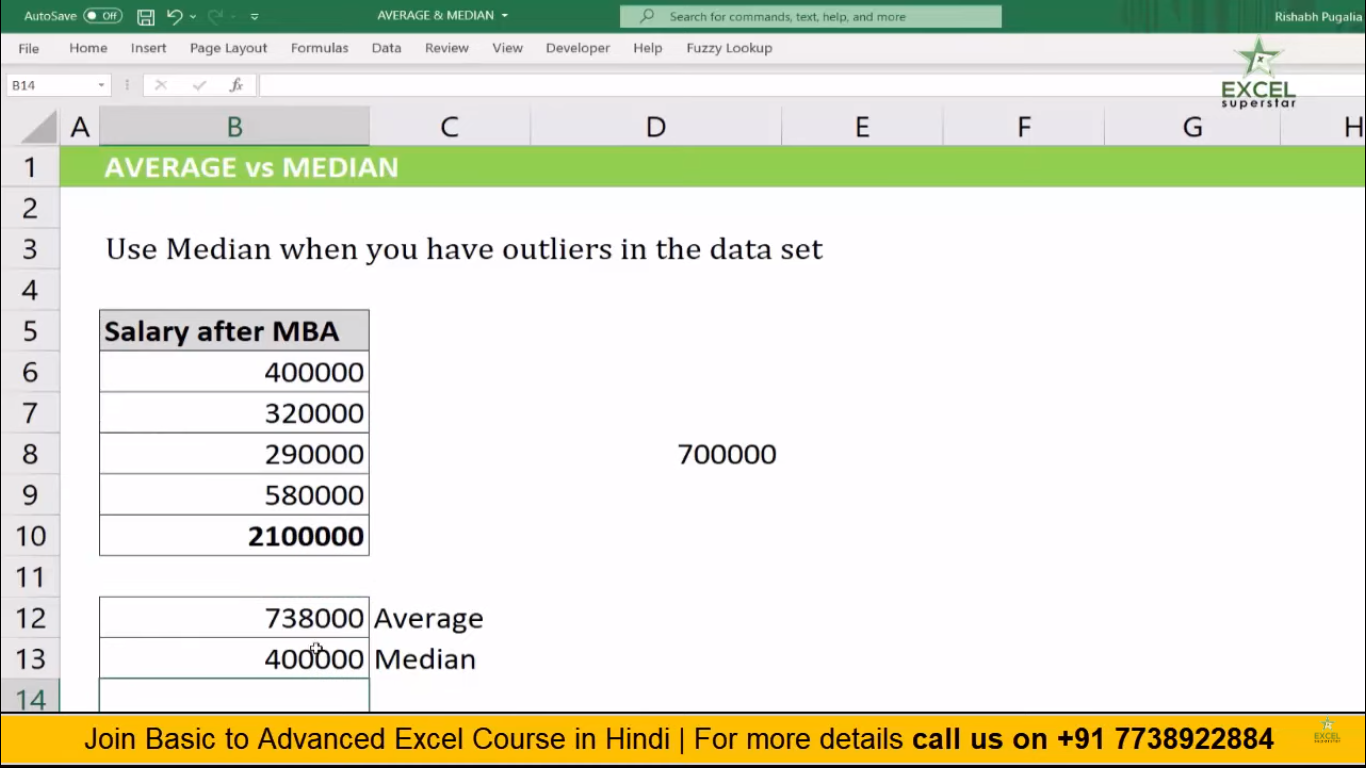Note – Outliers means which are different from the average data

4. Using MEDIAN Formula will give you a better information while making a decision. So whenever you see any outliers in the table then use MEDIAN formula along with the AVERAGE to see the difference of your answer in the given data.